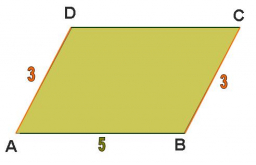# Parallelogram 64414

The parallelogram has side a = 58cm and diagonals u = 89cm, v = 52cm. Calculate the perimeter and area of this parallelogram.

o =  204.2836 cm
S =  2203.1082 cm2

### Step-by-step explanation:Did you find an error or inaccuracy? Feel free to write us. Thank you!

Tips for related online calculators
Need help calculating sum, simplifying, or multiplying fractions? Try our fraction calculator.
The Pythagorean theorem is the base for the right triangle calculator.